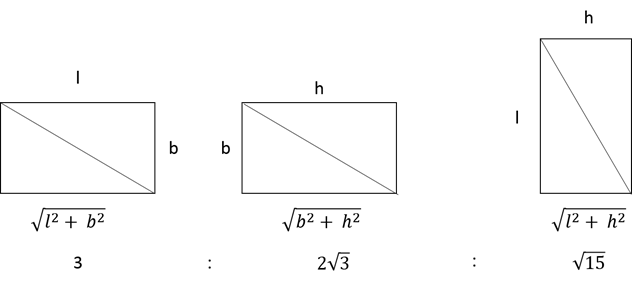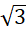# CAT 2019 Question Paper | Quants Slot 1

###### CAT Previous Year Paper | CAT Quants Questions | Question 27

CAT Geometry question that appears in the Quantitative Aptitude section of the CAT Exam broadly tests an aspirant on the concepts - Triangles, Circles, Quadrliaterals, Polygons & mixture of the above mentioned concepts. In CAT Exam, one can generally expect to get 4~6 questions from CAT Geometry. CAT Geometry is an important topic with lots of weightage in the CAT Exam. Make use of 2IIMs Free CAT Questions, provided with detailed solutions and Video explanations to obtain a wonderful CAT score.

Question 27 : If the rectangular faces of a brick have their diagonals in the ratio 3 : 2 $$sqrt{3}$ : $\sqrt{15}$, then the ratio of the length of the shortest edge of the brick to that of its longest edge is 1. 1 : $\sqrt{3}$ 2. 2 : $\sqrt{5}$ 3. $\sqrt{2}$ :$\sqrt{3}$ 4. $\sqrt{3}$ : 2 ## 🎉Get Upto ₹10,000 off on our CAT 23/24 courses.🎉Get flat ₹6,000 off on IPM courses.Valid till 30th September 2023! #### ★Register Now! ### Video Explanation ## Best CAT Coaching in Chennai #### CAT Coaching in Chennai - CAT 2022Limited Seats Available - Register Now! ### Explanatory AnswerFrom the given data, we know l2 + b2 = 9k --------$1)
b2 + h2 = 12k ------- (2)
l2 + h2 = 15k -------- (3)
Adding (1) , (2) and (3) we get
2(l2 + b2 + h2) = 36 k
l2 + b2 + h2 = 18k -----(4)
Subtracting (1), (2) , (3) from (4) we get
h2 = 9k
l2 = 6k
b2 = 3k
Thus, ratio of shortest edge to longest edge == 1:The question is "If the rectangular faces of a brick have their diagonals in the ratio 3 : 2 $$sqrt{3}$ : $\sqrt{15}$, then the ratio of the length of the shortest edge of the brick to that of its longest edge is " ##### Hence, the answer is 1 : $\sqrt{3}$ Choice A is the correct answer. ###### 🎉Get Upto ₹10,000 off on our CAT 23/24 courses.🎉Flat ₹6000 off on IPM courses. ###### Prepare for CAT 2023 with 2IIM's Daily Preparation Schedule ###### Know all about CAT Exam Syllabus and what to expect in CAT ###### Already have an Account? ###### CAT Coaching in ChennaiCAT 2023 Classroom Batches Starting Now! @Gopalapuram ###### Best CAT Coaching in Chennai Introductory offer of 5000/- Attend a Demo Class ###### Best Indore IPM & Rohtak IPM CoachingSignup and sample 9 full classes for free. Register now! ##### Where is 2IIM located? 2IIM Online CAT Coaching A Fermat Education Initiative, 58/16, Indira Gandhi Street, Kaveri Rangan Nagar, Saligramam, Chennai 600 093 ##### How to reach 2IIM? Mobile:$91) 99626 48484 / 94459 38484
WhatsApp: WhatsApp Now
Email: info@2iim.com